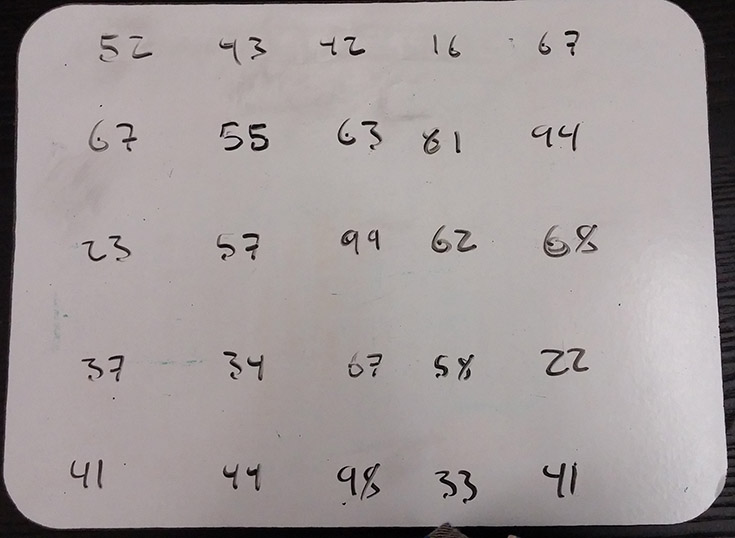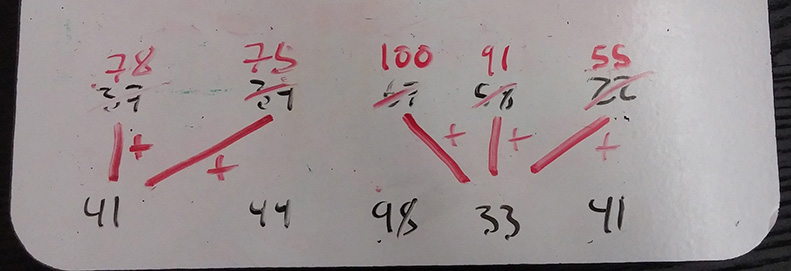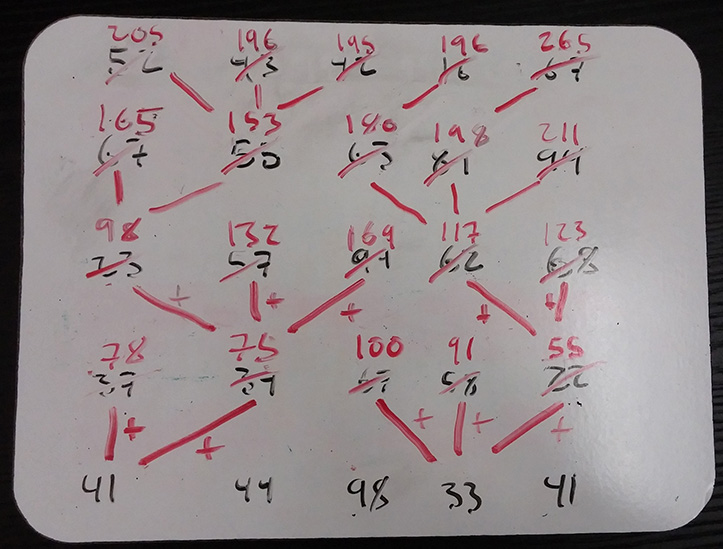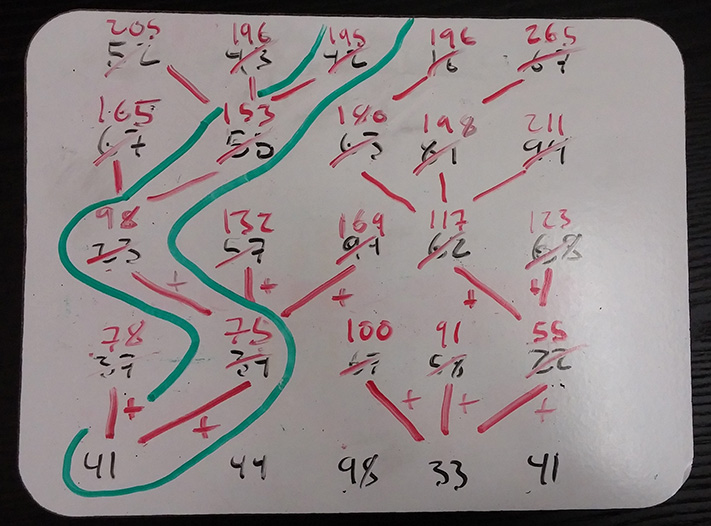# Dynamic programming illustrated – minimum path

This is the algorithm that helped me understand dynamic programming.

In the problem, you’re given a grid of numbers:Starting in the top row, you select a number, and add it to a running total. You look at the next row down, and pick the number either diagonal left, straight down, or diagonal right from your current choice, adding its value to the total. You then repeat the process from the selected number until you reach the bottom of the graph. The goal upon reaching the bottom is to have accumulated the lowest possible sum.

Try to think of the most naive way to do it. I’d select the lowest number on the top row, the lowest option on the next row down, and so on. This is the greedy solution. It’s easy to see the problem with the approach – taking a low value early on can lock you into a corridor full of choices much worse than you would have had by making a sacrifice for later gains.

We could find an optimal solution with a recursive approach, using a decision tree to try each and every possible path. This would always find the best route, but because it solves the same subproblems many times, it would be achingly slow.

Dynamic programming solves problems by breaking them into smaller subproblems, combining the results of each smaller solution to arrive at the solution to the whole. It seeks to solve each subproblem only once, avoiding the extra work caused by so-called subproblem overlap.

What are the subproblems in our particular problem? It helps to think about the work the recursive algorithm would do. For every node, it calculates the sum of that node with each of the nodes available in a path down the grid. So summing numbers is the bulk of the work here, but all we really need is the sum of the optimal subpath for each number in the grid. From there, we find the solution.

Start on the farthest-left value of the second to last row (37). Based on the rules in the problem definition, your potential moves if you had arrived here would be either 41 or 44. Select the lower number, 41, and add its value to 37’s. Cross out 37 and replace it with 78, the sum. Continue right, repeating the process for each number until you reach the end of the row.Work your way up from here, starting at the leftmost number of each row. Only consider the new sums in your calculations, not the crossed-out values.You’ll end up with the above. Every value except the last row has been replaced with a new value representing the sum of the best path to that point. We’ve now solved all the sub-problems from the bottom up, and the greedy solution from the top down will show us the optimal path.Notice that we avoided the trap of taking the early 16 on the first row, which would have led the path to clusters of high numbers and a much larger final sum.

I won’t show the code for this one, but I was originally exposed to this problem in an algorithms meetup hosted by Peter Hayes (github). His repo has a nice visual representation of the problem, as well as a solution implemented in JavaScript.

Written on August 2, 2014